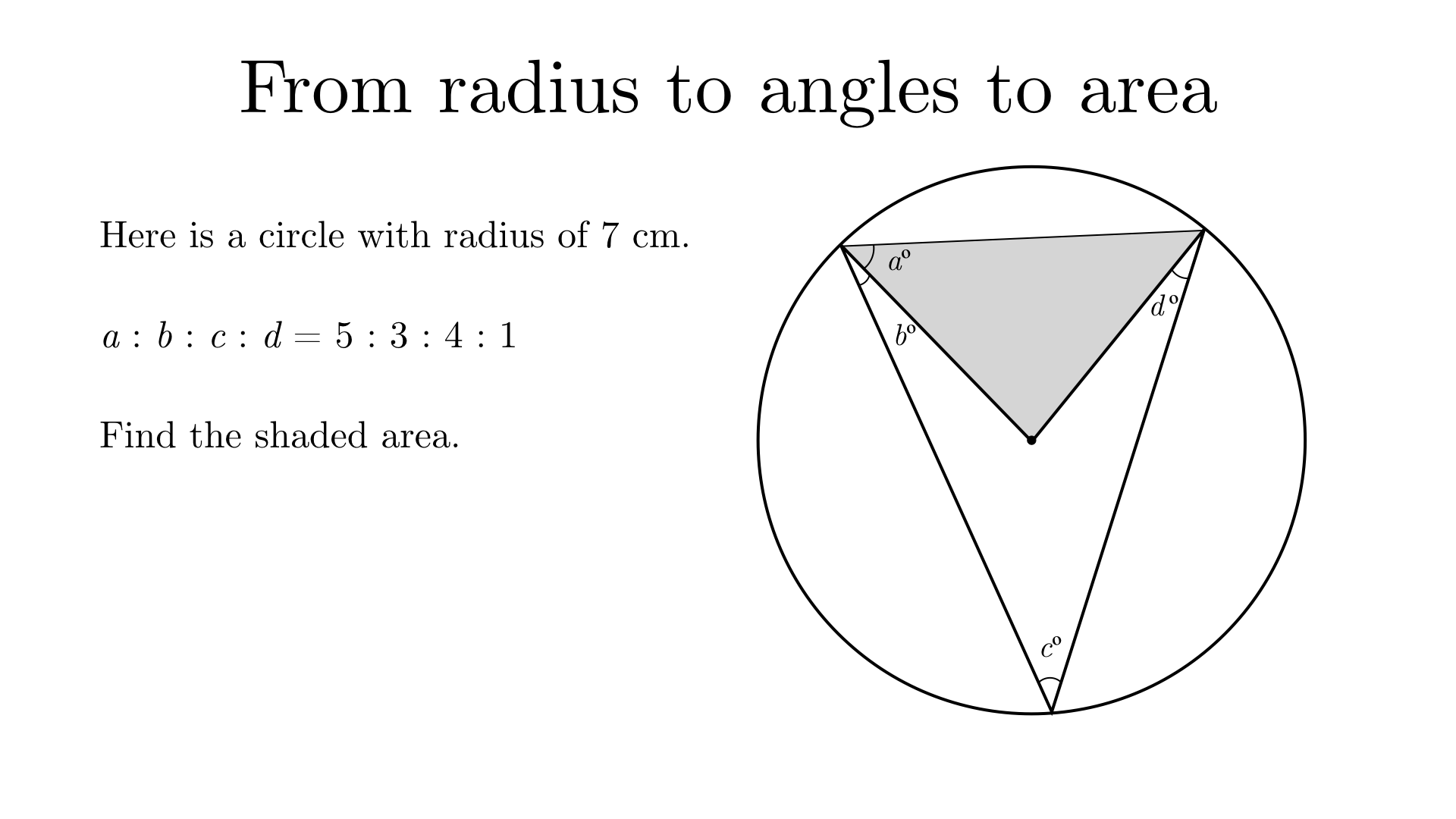Solution

The shaded triangle is isosceles as two of its sides are radii. Therefore, it has another angle of $$a$$º.

Consider the triangle with the 3 points on the circumference that has interior angles of $$(a+b)$$º, $$c$$º, and $$(a+d)$$º. These must sum to 180º so $$2a+b+c+d=180$$.

From the given ratio, we can say that $$2a:b:c:d = 10:3:4:1$$. Given that $$2a+b+c+d=180$$, we can share 180 in the ratio $$10:3:4:1$$ to find that $$2a = 100, b=30, c=40, d=10$$. Therefore, $$a = 50, b=30, c=40, d=10$$.

The angle at the centre of the circle in the shaded triangle is equal to double the angle $$c$$ at the circumeference, so is $$80$$º.

The area of the shaded triangle is $$\frac{1}{2}(7 \times 7)\text{sin}(80) = 24.1 \text{ cm}^{2}$$ to 3 significant figures.